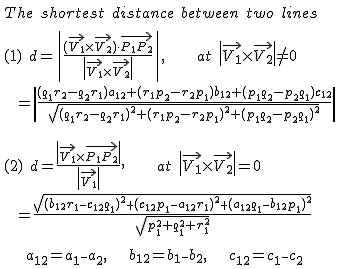## Shortest Distance Between Two Lines(Vectors) Calculator

 Line1 parallel to Vector V1(p1,q1,r1) through Point A(a1,b1,c1)
 Point A (,,) Vector V1 (,,)
 Line2 parallel to Vector V2(p2,q2,r2) through Point B(a2,b2,c2)
 Point B (,,) Vector V2 (,,)
 Shortest distance between two lines

The online calculator to find the shortest distance between given two lines in space.

### Formula

Line1 parallel to Vector V1(p1,q1,r1) through Point A(a1,b1,c1), Line2 parallel to Vector V2(p2,q2,r2) through Point B(a2,b2,c2)Thinkcalculator.com provides you helpful and handy calculator resources.